# What is a Flowchart

## What is a Flowchart?

In this article, I will give you a brief introduction to the flowchart. Flow charts were used at the time when monolithic programming was there. Later on, when procedural programming came, the usage of flow charts was little reduced. And today we are following object-oriented programming or aspect-oriented programming, so the usage of flowcharts is very much less.

##### What is a Flowchart?

A flowchart is used for showing the flow of control in a program and the sequence of steps involved in a hierarchical manner. It is basically a diagrammatic representation of an algorithm, workflow, or process.

So, if a program is very big then it is very difficult to figure out how the flow of program is, Flow charts are useful for understanding the program, instead of one is reading the program and understanding, he can see the flow chart and understand how the program is working.

It is just like if you talk about electrical wiring in a home. Then from where the wires or the cables are moving through the walls. If you have a plan then you can know where exactly they are flowing and where the important points are, everything you can know. Otherwise, if there is any problem with the wiring, then you have to dig the whole wall to find out the problem. If there is a proper plan then you can understand. So before laying the wire or pulling the wires we will make a plan. The same way before writing the program we make a flowchart.

So based on the flow chart we will write the program. This will help us to understand the program.

##### Use of Flowchart

Flowcharts were highly used at the times of Monolithic Programming. Then later on when the concept of Procedural Programming came into practice, the usage of flowcharts was little reduced.

##### Steps in the flowchart:

Usually, when we are using a flow chart for the program, it consists of three steps:

1. Input
2. Process
3. Output

We will call it like this. First, it takes some input. Then it will process. Then it will give output. So, any procedure you take will have similar steps. For example, preparing a dish. Input is the ingredients. That process is the process of making a dish and output is the dish ready. If you take a chemistry experiment that is done usually in laboratories will have input means chemicals and the vessels or instruments whatever you need. Then the process of what you will do with that and then it gets done successfully. So, every procedure will have these 3 things and the program is also used to look like this.

##### Elements of Flowchart:

Now let us look at the elements of the flow chart. The following image shows the different elements of a flowchart.

Terminal: The oval symbol indicates Start, Stop and Halt in a program’s logic flow. A pause/halt is generally used in programming logic under some error conditions. The terminal is the first and last symbol in the flowchart.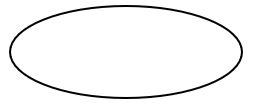Input/Output: A parallelogram denotes any function of input/output type. Program instructions that take input from input devices and display output on output devices are indicated with a parallelogram in a flowchart.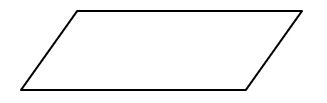Processing: A box represents arithmetic instructions. All arithmetic processes such as addition, subtraction, multiplication, and division are indicated by the action/process symbol.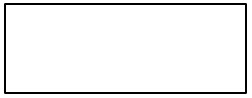Decision: Diamond-shaped symbol represents a decision point. Decision-based operations such as Yes/No, question, or True/False are indicated by diamond shape in the flowchart.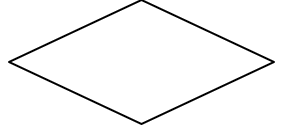Flow lines: Flow lines indicate the exact sequence in which instructions are executed. Arrows represent the direction of flow of control and the relationship among different symbols of the flowchart.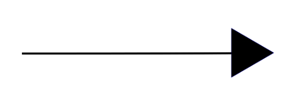Now let us draw a few flow charts and try to understand the idea of how flowcharts are used and how they are useful for writing the programs.

##### Flowchart for adding two numbers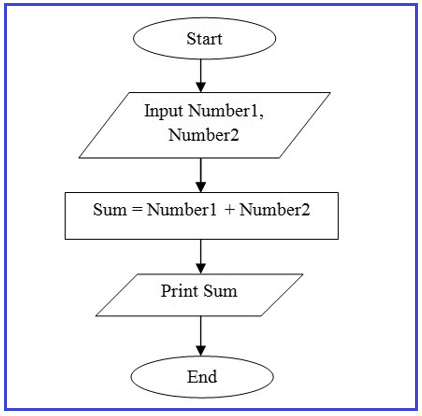Step 1: Start.
Step 2: Declare variables Number1 and Number2.
Step 3: Read values Number1 and Number2.
Step 4: Add Number1 and Number2 and store the result in Sum. (Sum = Number1 + Number2).
Step 5: Display Sum.
Step 6: Stop.

##### Flowchart for Greater in two numbers

Now I will draw a flowchart for finding the greater number in two numbers. If you have two numbers then which one is the greater, we will find out by creating the flowchart. So here it will be the same, we need to take two numbers and store them into the variables let say, a and b. Then from a and b, we will find out which one is greater. Whichever is greater we will print that greater number. This is the step that is described in the below flowchart.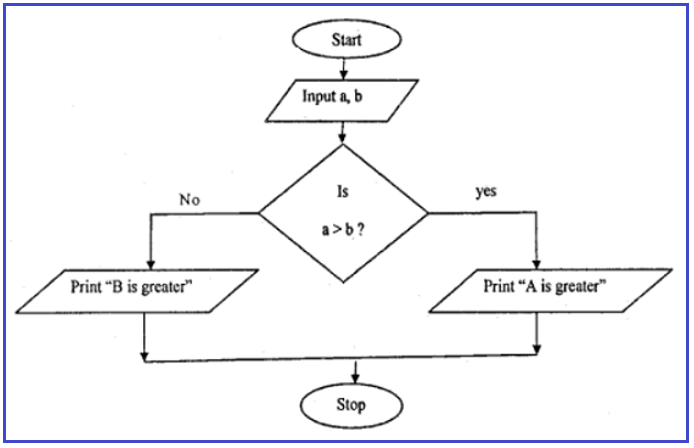‌Step 1: Start.
‌Step 2: Declare variables a and b.
‌Step 3: Read values a and b.
‌Step 4:  If a>b then        /*Checking */
Display “A is greater”
Else
Display “B is greater”.
‌Step 5:  Stop

##### Flowchart for Print numbers from 1 to 10.

Now we will see one more flowchart for printing numbers from 1 to 10. So, for printing numbers we have to do two things, one is print and the second one is counting like print 1, print 2 and print 3. The following flowchart shows how to print 1 to 10.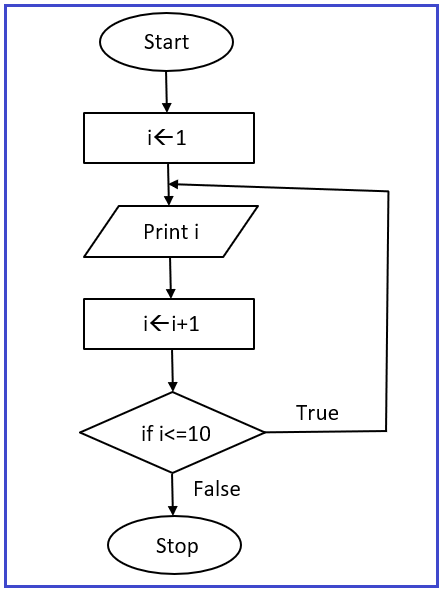Step 1: Start.
Step 2: In processing initialize i to 1.
Step 3: Print i (i.e., 1).
Step 4: Add 1 in i and store the result in i. /* so, it will increase the value of i by one */
Step 5: if i<=10 /*Checking */
Print i again and increment
Otherwise
Step 6: Stop.

In the next article, I am going to discuss Steps for Program Development and Execution. Here, in this article, I try to explain What is Flowchart and I hope you enjoy this What is Flowchart article.